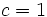# Baer invariants and extension theory for Klein four-group

## Contents

This article gives specific information, namely, Baer invariants and extension theory, about a particular group, namely: Klein four-group.
View Baer invariants and extension theory for particular groups | View other specific information about Klein four-group

This article discusses the Baer invariants and the corresponding extension theory for the Klein four-group, which we denote$V_4$, corresponding to a number of subvarieties of the variety of groups.

## Nilpotent multipliers and nilpotent extension theory

### Nilpotent multipliers

We can use the fact that nilpotent multiplier of abelian group is graded component of free Lie ring to compute the nilpotent multipliers. The$c^{th}$ nilpotent multiplier$M^{(c)}(V_4)$ is explicitly given as an elementary abelian 2-group of rank given by the formula for dimension of graded component of free Lie algebra:$M^{(c)}(V_4) = (\mathbb{Z}/2\mathbb{Z})^j$

where:$j = \frac{1}{c + 1} \sum_{d \mid (c + 1)} \mu(d)2^{(c + 1)/d}$

where$\mu$ is the Mobius function.

The explicit formulas are below. Note that the case$c = 1$ gives us the Schur multiplier, also described as the second homology group for trivial group action with integer coefficients (see also group cohomology of Klein four-group):$c$$c + 1$ Formula calculating rank of$M^{(c)}(V_4)$ Rank of$M^{(c)}(V_4)$ Group$M^{(c)}(V_4)$
1 2$\frac{2^2 - 2}{2}$ 1 cyclic group:Z2
2 3$\frac{2^3 - 2}{3}$ 2 Klein four-group
3 4$\frac{2^4 - 2^2}{4}$ 3 elementary abelian group:E8
4 5$\frac{2^5 - 2}{5}$ 6 elementary abelian group:E64
5 6$\frac{2^6 - 2^3 - 2^2 + 2}{6}$ 9 elementary abelian group:E512

### Extension theory

It is possible to construct extension that realize these Baer invariants; however, the constructions are not necessarily of the same order as the corresponding Lie ring constructions (which provide a lower bound).

Consider the case$c = 2$. We want to construct a group$G$ such that$G/Z^2(G)$ (where$Z^2(G)$ denotes the second center of$G$) is isomorphic to the Klein four-group and$\gamma_3(G)$ is also isomorphic to the Klein four-group (because that is the value of$M^{(2)}$ for the Klein four-group).

If we consider the quotient of the free Lie ring$F$ on$V_4$ by$\gamma_4(F)$, we obtain a class three Lie ring of order 32, with a gradation and where the ranks of the graded pieces are 2, 1, and 2 (the numbers 1 and 2 are respectively the ranks of the Schur multiplier and the 2-nilpotent multiplier). There is no corresponding group of order 32.

There do exist some groups among the groups of order 64, however. The second parts of their group IDs in the SmallGroup library are 8, 9, 10, 11, 12, 13, 14 (name of Hall-Senior family?).